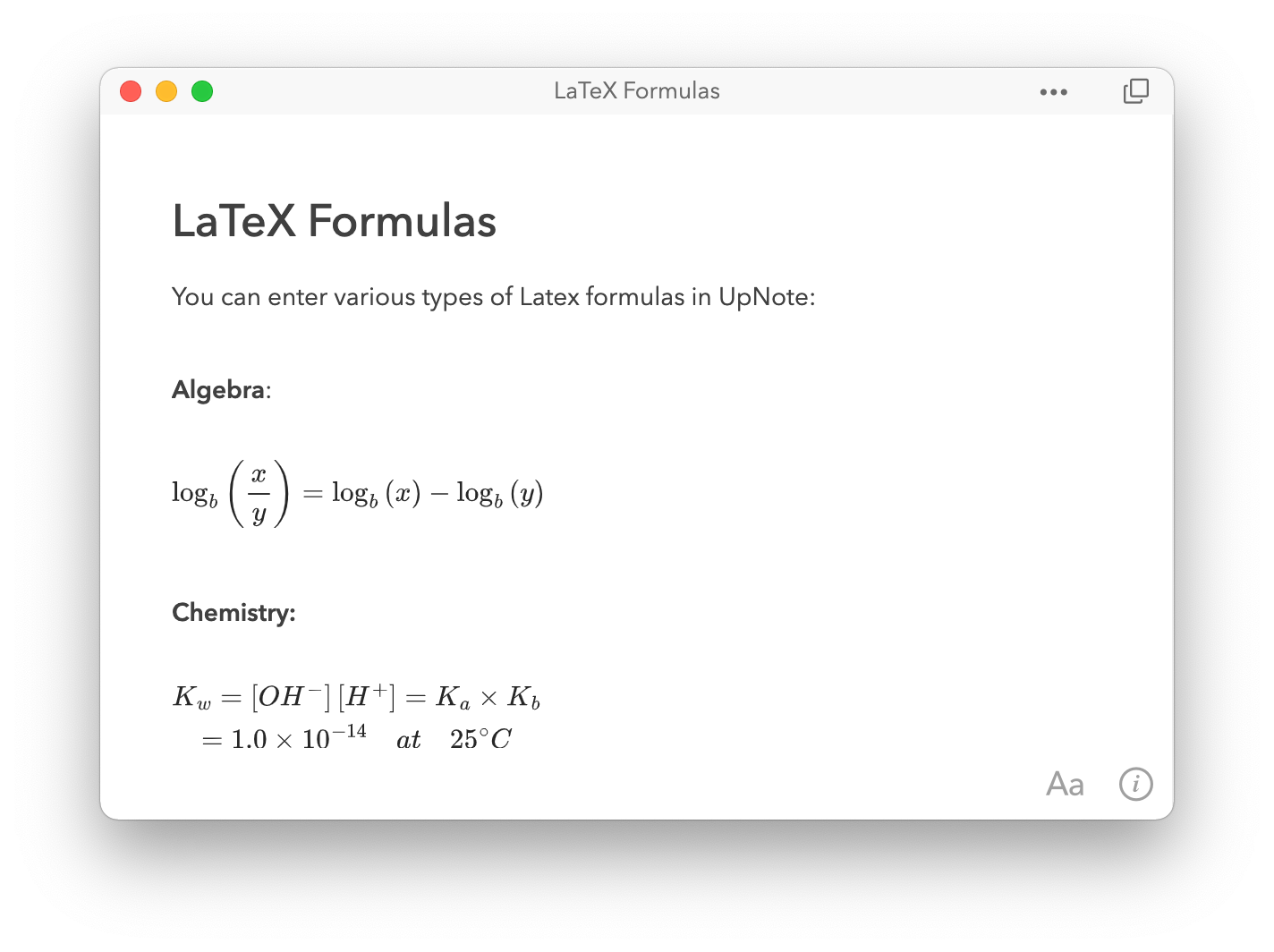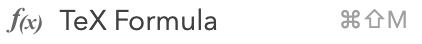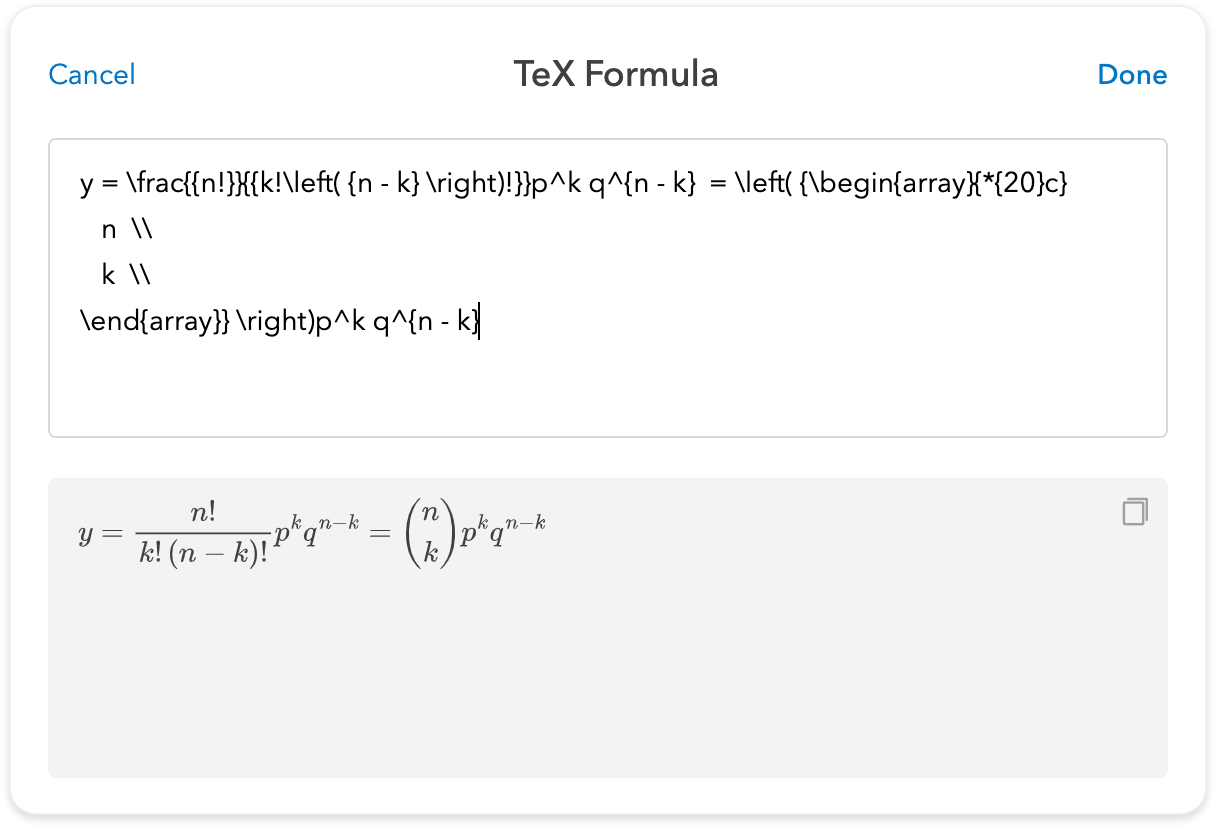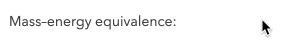# Insert LaTeX formulas

LaTeX formulas are popular for writing math, physics and chemistry equations.To insert LaTeX formulas, you can click or tap the TeX formula icon in the formatting bar or use the shortcut Ctrl/Cmd + Shift + M on your desktop app.When you type the formula, UpNote automatically display the preview in the bottom box. It can also help you check for syntax error so you can correct the formula when needed.You may insert an in-line formula or quickly convert a text to equation when enclosing the text in  delimiters.It’s easy to edit the formula by simply clicking on the formula and editing it in the input box.
There are a few resources to help you quickly master LaTex formulas, for example Overleaf or Wikibooks.
On a technical note, the LaTeX formulas are rendered with MathJax library. A huge thanks to MathJax team for the excellent library. MathJax supports most mathematical notation in LaTeX. You might view their documentation for the full list.